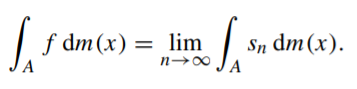# Lebesgue Integration: Overview / Simple Definition

Lebesgue integrals are a powerful form of integration that can work with the most pathological of functions, including unbounded functions and highly discontinuous functions.

## Difference Between Riemann Integration and Lebesgue Integration

Riemann integrals work by subdividing the domain into a number of piecewise constant functions for each sub-interval. Lebesgue integration works by subdividing the range instead. An intuitive example of the difference between the two is given in this analogy by Chapman (2010):

Riemann and Lebesgue add up a group of coins with different face values. Riemann adds up each of the coin’s face values, one by one. Let’s say he picks up a dime, a nickel and then a penny: he’ll count 10 + 5 + 1…. continuing until all of the coins have been added, individually. Then he’ll give his total. In contrast, Lebesgue first sorts the coins into piles of dimes, nickels and pennies. He counts each pile, then sums up the totals from each pile.

When Lebesgue sorts the coins into piles, he’s partitioning the value axis (i.e. the axis with the coin’s numerical values) and taking preimages—sets of function arguments that correspond to a subset in the range. These preimages are the fundamental building blocks of Lebesgue integration.

The basic procedure (Tao, 2010) is:

1. Subdivide the function’s range into a finite number of segments.
2. Construct a simple function by using a function with values that are the same finitely many numbers.
3. Keep on adding points in the range of the original function, taking the limit as you go.

## Formal Definition of the Lebesgue Integral

The Lebesgue integral can be formally defined as (Wojas & Krupa, 2017):Where:

• sn : A ↦ ℝ is a nondecreasing sequence of nonnegative simple measurable functions, the limit of which is limn→∞ sn(x) = f(x) for every x ∈ A. (note: A is a Lebesgue measurable subset of ℝ).

Note that for this particular definition, the order of sn is not important.

## References

Chapman, C. (2010). Real Mathematical Analysis (Undergraduate Texts in Mathematics). Springer New York.
Hunter, J. Riemann Integral. Retrieved January 14, 2019 from: https://www.math.ucdavis.edu/~hunter/m125b/ch1.pdf
Kestelman, H. “Lebesgue Integral of a Non-Negative Function” and “Lebesgue Integrals of Functions Which Are Sometimes Negative.” Chs. 5-6 in Modern Theories of Integration, 2nd rev. ed. New York: Dover, pp. 113-160, 1960.
Papoulis, A. Probability, Random Variables, and Stochastic Processes, 2nd ed. New York: McGraw-Hill, p. 141, 1984.
Tao, T. (2010). 245A, Notes 2: The Lebesgue integral. Retrieved January 14, 2020 from: https://terrytao.wordpress.com/2010/09/19/245a-notes-2-the-lebesgue-integral/
Wojas, W. & Krupa, J. Familiarizing Students with Definition of Lebesgue Integral: Examples of Calculation Directly from Its Definition Using Mathematica. Math.Comput.Sci. (2017) 11:363–381
DOI 10.1007/s11786-017-0321-5

CITE THIS AS:
Stephanie Glen. "Lebesgue Integration: Overview / Simple Definition" From StatisticsHowTo.com: Elementary Statistics for the rest of us! https://www.statisticshowto.com/lebesgue-integration-overview-simple-definition/